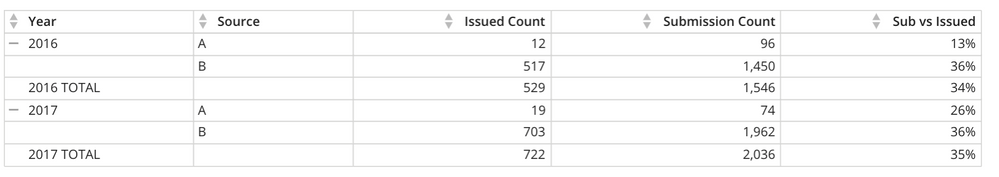# Table Subtotal not calculating beast mode

Hi, I have a table with below records. The subtotal for % column is simply adding the values instead of applying the beast mode calculation at the subtotal level. 2016 Total row % should be 34% instead of 49% and 2017 should be 35% instead of 62%. Has something changed with recent releases?

 Year Source Submission Count Issued Count Sub vs issued 2016 A 96 12 13% B 1450 517 36% 2016 Total 1546 529 49% 2017 A 74 19 26% B 1962 703 36% 2017 Total 2036 722 62%

• interesting.  I'll be honest I didn't test this, but I'm pretty confident the pivot table is acting as expected.

In your math, ignoring the adjustment to change units from year to months, you've said

"for each row, take issued count and divide it by submission count THEN take the sum."

what i believe you want is:  "take total issued count divided by total submission count."

In that case, your base math should be:

sum(issued count) / sum(submission count)

VERIFY THAT THIS MATH IS CORRECT

IF SO, then to accommodate for your adjustment for the current year, nest your CASE statement INSIDE the SUMs.

sum(

issued count / case when year ... then 12 else month(curdate()) end

) /

sum(

submission count / case when year ... then 12 else month(curdate()) end

Hopefully that makes sense.

@user016969 wrote:

Hi, My beast mode calc is -

case
when `Year` != YEAR(CURDATE())
then (`Issued Count`/ 12) / (`Submission Count`/ 12)
else (`Issued Count`/ MONTH(CURDATE()))/ (`Submission Count`/ MONTH(CURDATE()))
end

Jae Wilson
Check out my 🎥 Domo Training YouTube Channel 👨‍💻

**Say "Thanks" by clicking the ❤️ in the post that helped you.
**Please mark the post that solves your problem by clicking on "Accept as Solution"

• oh interesting!  what's your beast mode calc? (this might be something to raise to support)

Jae Wilson
Check out my 🎥 Domo Training YouTube Channel 👨‍💻

**Say "Thanks" by clicking the ❤️ in the post that helped you.
**Please mark the post that solves your problem by clicking on "Accept as Solution"
• Hi, My beast mode calc is -

case
when `Year` != YEAR(CURDATE())
then (`Issued Count`/ 12) / (`Submission Count`/ 12)
else (`Issued Count`/ MONTH(CURDATE()))/ (`Submission Count`/ MONTH(CURDATE()))
end

• Thanks but 'Subtotal' record should perform that logic. I removed all case statement and simply made the beast mode with 'Issued count / submission count'. Still, subtotal record does not calculate this baest mode and simply adds values. e.g. 2016 Total record should calculate 529/1546, but what it does is 13 (% of A) + 36 (% ofgiving 49. I observed that at column level there is 'Subtotal/Total' option that is by default set to 'Sum'. My guess is that this sum overrides beast mode calculation for subtotal row. I think the column level drop down should have additional option where user wants beast mode to be calculated instead of listed calculations (Max, Min, Avg, Count and Sum).

• May be I should clarify that the 'Total' records are calculated using 'Subtotal' chart property and Sub vs. Issued is a beast mode calc. Original data is as follows:

 Year Source Submission Count Issued Count 2016 A 96 12 2016 B 1450 517 2017 A 74 19 2017 B 1962 703

After beast mode addition it is -

 Year Source Submission Count Issued Count Sub vs issued 2016 A 96 12 13% B 1450 517 36% 2017 A 74 19 26% B 1962 703 36%

After adding chart property of subtotal it becomes as noted in original question. So when added subtotal, 'Sub vs Issued' column should also calculate beast mode for the subtotal record.

• @user016969 - This is because how the Sub Total option works. It doesn't know anything about your beast mode but rather it's just adding up all of the previous row values. This is why you're getting 13% + 36% = 49% rather than 529 / 1546 = 34%. It's because of the order of operations used to calculate your percentage.

Put in more mathematical terms this is how the Sub Total is processing your data:

(12 / 96 ) + (517 / 1450)

( 12 + 517 ) / ( 96 + 1450 )

This is why @jaeW_at_Onyx metioned wrapping your fields in a SUM() aggregation so that the addition will be done first before the division.

`SUM(`Issued Count`) / SUM(`Submission Count`)`

Utilizing that beast mode gets you the expected results: